Population splitting using Probplot

Input data are soil analyses from Daisy property, Montana (-80 mesh fraction, aqua-regia digestion, 0.5 gram aliquot). First 27 lines of input data file (ASCII) are as follows:

247 24 (Number of samples, number of variables)

N 0 2 4 6 6 6 6

“N” indicates case or sample number type code is numeric; A would indicate it was alphanumeric. “0” indicates length of alphanumeric case number (not applicable in this case). “2” defines the number of

numeric fields immediately after the case number that are not to be analyzed. ”4” defines the number of lines which contain the data from each case; and “6” defines the number of variables on each of the 4 lines.

Daisy Creek Soil Grid

(Name of data set. This will be reproduced on all graphical output)

CU     PPM (Names and units of measurement of input variables)

PB     PPM

ZN     PPM

AG     PPM

NI     PPM

CO     PPM

MN     PPM

FE     PCT

AS     PPM

SR     PPM

SB     PPM

SI     PCT

V     PPM

CA     PCT

P     PCT

LA     PPM

CR     PPM

MG     PCT

BA     PPM

TI     PCT

B     PPM

AL     PCT

NA     PCT

K     PCT

(First line of data follows)

7020  1550  1650   553.00    19.00    94.00     1.10    15.00   11.00

770.00     2.02     3.00    16.00     2.00    .06

31.00      .16      .13    12.00    10.00     .22

330.00      .11     8.00     2.45      .02      .13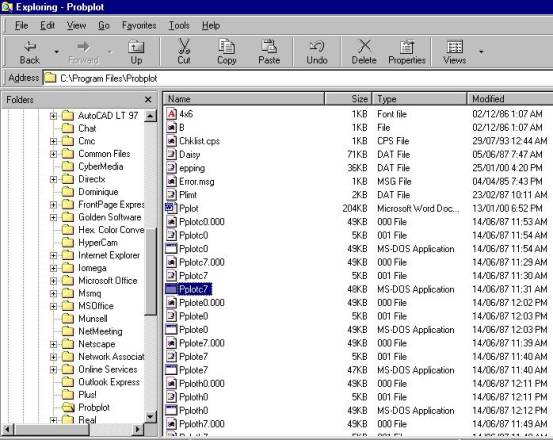Step 1: Run PROBLOT from Windows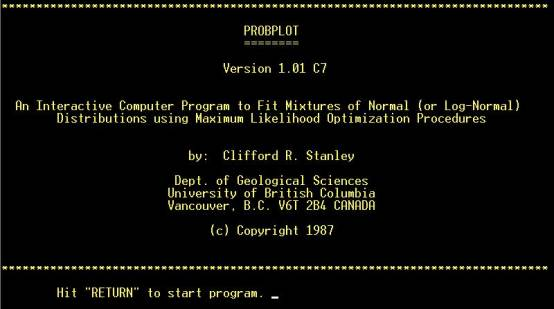Introductory screen. No action required (except hitting “RETURN”)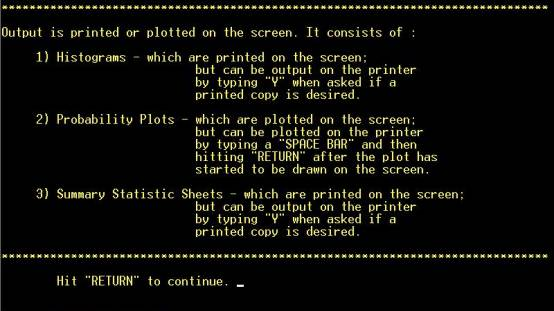Output summary. No action required (except hitting “RETURN”)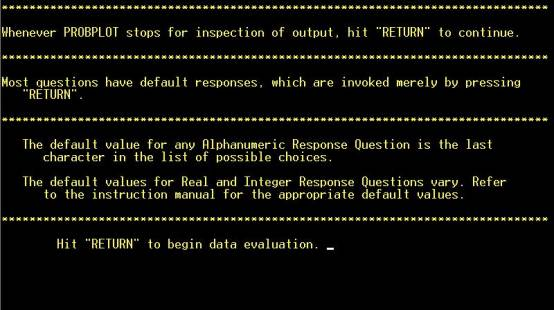No action required (except hitting “RETURN”)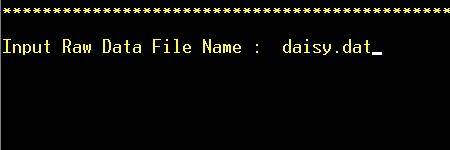Step 2: Enter input data file name (for correct formatting of data in file, see above)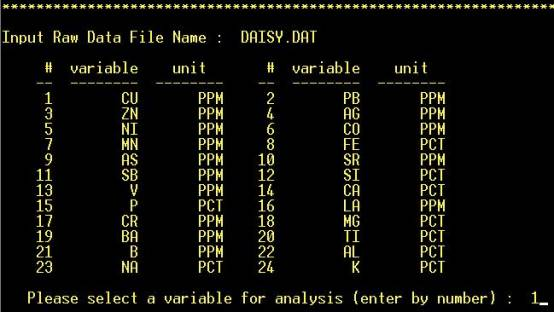Step 3: Variable is selected by number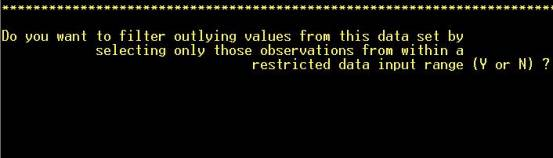Step 4: Edit outliers from data, if desired. NOTE: Hot key (not necessary to hit “RETURN”)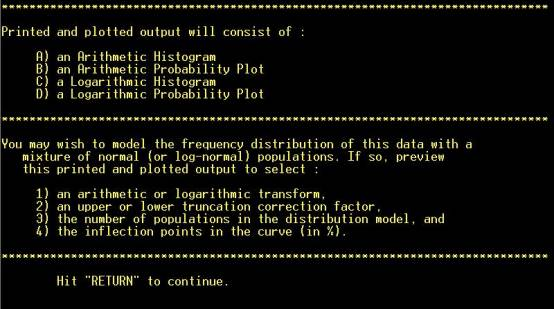No action required (except hitting “RETURN”)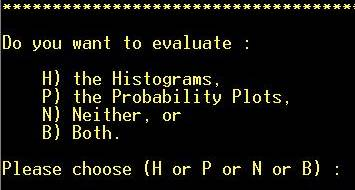Step 5: Choose evaluation method. “P” will be chosen in this case. NOTE: Hot key (not necessary to hit “RETURN”)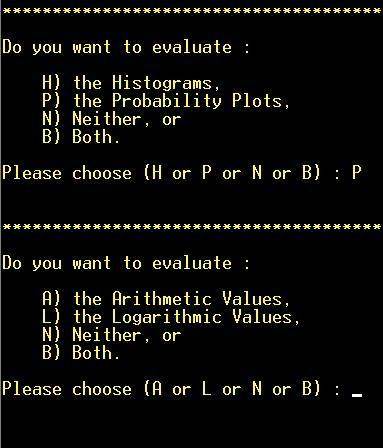Step 6: Choose transformation method. “L” will be chosen in this case. NOTE: Hot key (not necessary to hit “RETURN”)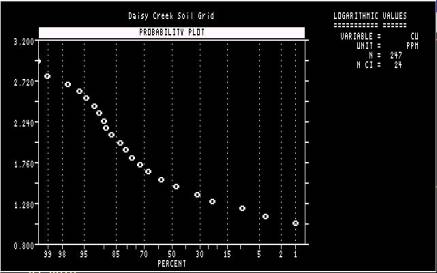First cumulative frequency plot. Advisable to print this out, or do a screen capture (PrtSc) and copy into MS-Paint, so that it can be referred back to later. NOTE: X-axis scaled in reverse order. The plot will disappear (and the program continue) if “RETURN” is hit.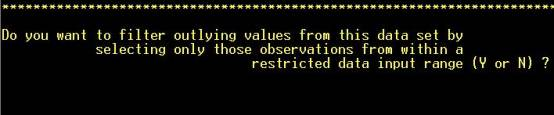Step 7: Edit outliers from data, if desired. NOTE: Hot key (not necessary to hit “RETURN”)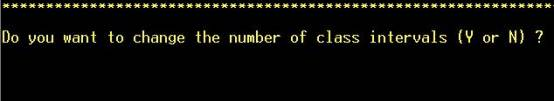Step 8: Change number of class intervals, if desired. NOTE: Hot key (not necessary to hit “RETURN”). If “Y” is answered to either this or the preceding question, a new graph will be drawn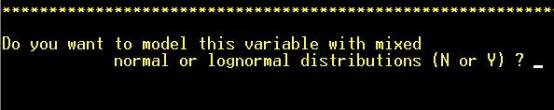Step 9: The important question being asked here is “Do you want to model this variable with mixed distributions?”. Answering “N” will result in the program starting again with a new variable.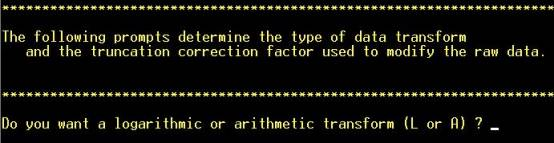Step 10: Choose whether to transform the data. Logarithmic transforms are commonly required for trace-element geochemical data but ultimately this depends on the frequency distribution of this element in this data set. NOTE: Hot key (not necessary to hit “RETURN”).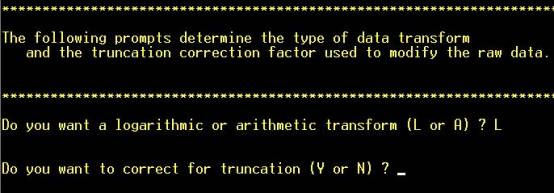Answering “Yes” is appropriate if a significant proportion of the analyses are “undetectable” (not in this case). NOTE: Hot key (not necessary to hit “RETURN”).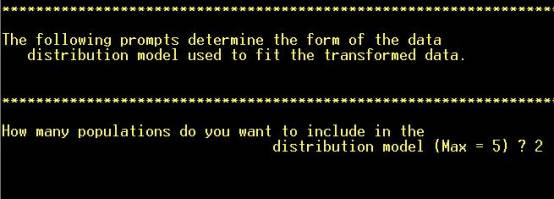Step 11: Choose how many subpopulations to model (inspect the cumulative frequency plot)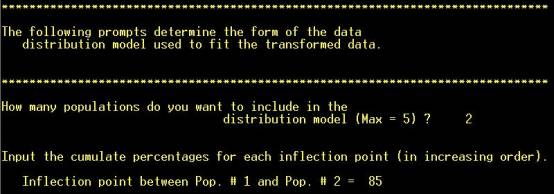Step 12: Read off an approximate cutoff between the subpopulations from the X-axis of the cumulative frequency plot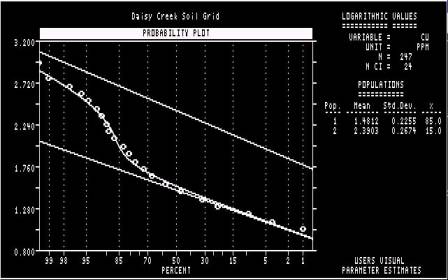The samples falling either side of the cutoff selected in Step 11 are assigned to two different sub-populations. The curve represents the recombination of the two modelled sub-populations, and the closeness of its correspondence to the observed distribution represented by the points is an indication of how good the cutoff estimate (of 85%) was. The program will continue if “RETURN” is hit.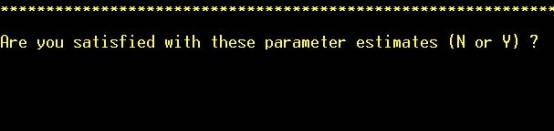Step 13: A decision is taken whether to seek a better cutoff. NOTE: Hot key (not necessary to hit “RETURN”).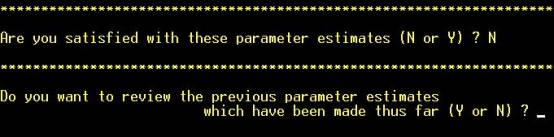Step 13: If “Y” is hit, all previous estimates of the number of sub-populations, and the cutoffs that separate them, will be listed on the screen.  NOTE: Hot key (not necessary to hit “RETURN”).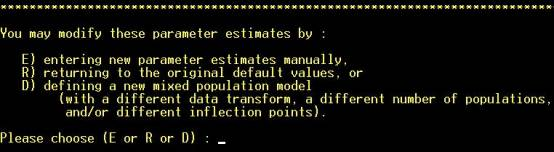Step 14: To enter a new estimate of the number of sub-populations, and the cutoffs that separate them, hit “D”. If “E” is hit, the program will prompt the user for estimates of the mean and standard deviation of the subpopulations. NOTE: Hot key (not necessary to hit “RETURN”).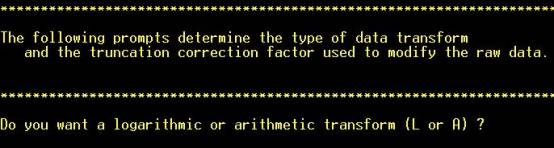Step 15: Step 10 is repeated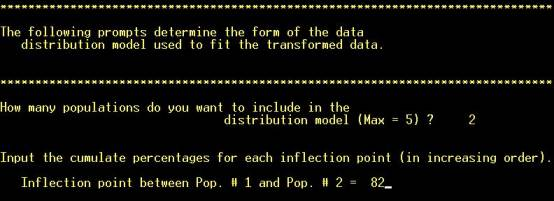Step 16: Step 12 is repeated, with a different cutoff between the two sub-populations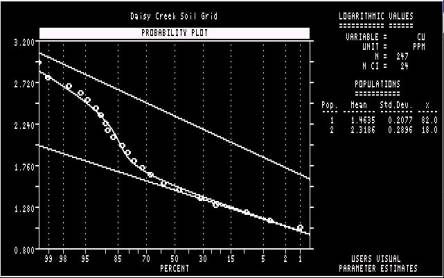Step 17: Once again, the correspondence between the modelled subpopulations (curved line) and the observed distribution (points) is evaluated.Step 18: As in Step 13, a decision is taken whether to seek a better cutoff. NOTE: Hot key (not necessary to hit “RETURN”). In this case, “Y” will be hit. NOTE: Hot key (not necessary to hit “RETURN”).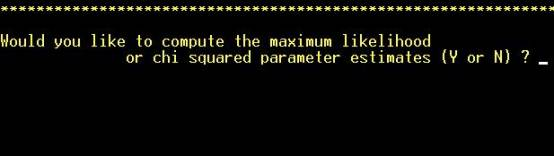Step 19: If desired, Probplot itself will seek an optimal split. This is not usually necessary if there are only two subpopulations but more desirable if more than two are identified. NOTE: Hot key (not necessary to hit “RETURN”).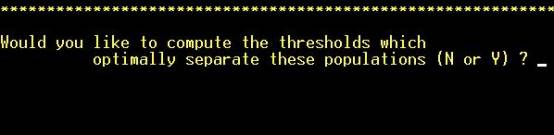Step 20: The “thresholds” in question refer to the mean plus two standard deviations of each of the modelled populations represented by the straight, inclined lines. They do not refer to the cutoffs, in analytical units, that separate the subpopulations. NOTE: Hot key (not necessary to hit “RETURN”).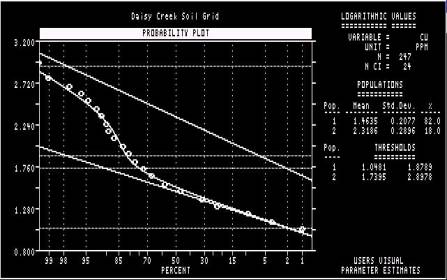Thresholds are calculated.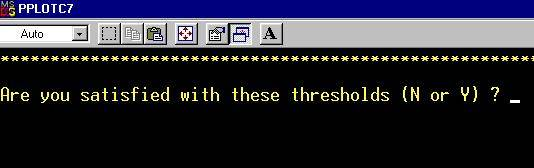Step 21 (it is necessary to execute the remaining 4 steps in order to exit from the program). NOTE: Hot key (not necessary to hit “RETURN”).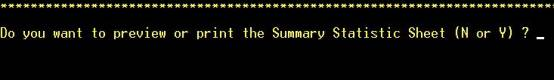Step 22 NOTE: Hot key (not necessary to hit “RETURN”).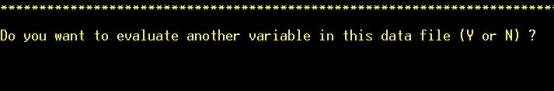Step 23 NOTE: Hot key (not necessary to hit “RETURN”).

Go to Directory Page